# Stiffness, Stability, and Automatic Differentiation

##### January 18th, 2020

In this lecture we will start discussing how "error" in a local sense is not the full story behind convergence, and showcase how stiff systems arise, are solved, and use this as an introduction for understanding automatic differentiation choices.

## Theory and Numerics of Stiff ODEs

The literature behind stiff ordinary differential equations is very deep and dense, and so we'll dive right in with a very practical approach. One of the leading figures of numerical differential equations, Lawarence Shampine described a stiff ODE as one where an explicit method is slower than an implicit method. In practice this is quite a good operational definition, but let's look at some theory as to why this could be a case, what an implicit method is, and what user choices need to be done to make solving difficult differential equations practical.

### Stability of a Method

Simply having an order on the truncation error does not imply convergence of the method. The disconnect is that the errors at a given time point may not dissipate. What also needs to be checked is the asymtopic behavior of a disturbance. To see this, one can utilize the linear test problem:

$u' = \alpha u$

and ask the question, does the discrete dynamical system defined by the discretized ODE end up going to zero? You would hope that the discretized dynamical system and the continuous dynamical system have the same properties in this simple case, and this is known as linear stability analysis of the method.

As an example, take a look at the Euler method. Recall that the Euler method was given by:

$u_{n+1} = u_n + \Delta t f(u_n,p,t)$

When we plug in the linear test equation, we get that

$u_{n+1} = u_n + \Delta t \alpha u_n$

If we let $z = \Delta t \alpha$, then we get the following:

$u_{n+1} = u_n + z u_n = (1+z)u_n$

which is stable when $z$ is in the shifted unit circle. This means that, as a necessary condition, the step size $\Delta t$ needs to be small enough that $z$ satisfies this condition, placing a stepsize limit on the method.### Interpretation of the Linear Stability Condition

To interpret the linear stability condition, recall that the linearization of a system interprets the dynamics as locally being due to the Jacobian of the system. Thus

$u' = f(u,p,t)$

is locally equivalent to:

$u' = \frac{df}{du}u$

You can understand the local behavior through diagonalizing this matrix. Therefore, the scalar for the linear stability analysis is performing an analysis on the eigenvalues of the Jacobian. The method will be stable if the largest eigenvalues of df/du are all within the stability limit. This means that stability effects different

### Implicit Methods

If instead of the Euler method we defined $f$ to be evaluated at the future point, we would receive a method like:

$u_{n+1} = u_n + \Delta t f(u_{n+1},p,t+\Delta t)$

in which case, for the stability calculation we would have that

$u_{n+1} = u_n + \Delta t \alpha u_n$

or

$(1-z) u_{n+1} = u_n$

which means that

$u_{n+1} = \frac{1}{1-z} u_n$

which is stable for all $Re(z) < 0$ a property which is known as A-stability. It is also stable as $z \rightarrow \infty$, a property known as L-stability. This means that for equations with very ill-conditioned Jacobians, this method is still able to be use reasonably large stepsizes and can thus be efficient.### Stiffness and Timescale Separation

From this we see that there is a maximal stepsize whenever the eigenvalues of the Jacobian are sufficiently large. It turns out that's not an issue if the phonomena we fast to see is fast, since then the total integration time tends to be small. However, is we have some equations with both fast modes and slow modes, like the Robertson equation, then it is very difficult because in order to resolve the slow dynamics over a long timespan, one needs to ensure that the fast dynamics do not diverge. This is a property known as stiffness. Stiffness can thus be approximated in some sense by the condition number of the Jacobian. The condition number of a matrix is its maximal eigenvalue divided by its minimal eigenvalue and gives an rough measure of the local timescale separations. If this value is large and one wants to resolve the slow dynamics, then explict integrators, like the explicit Runge-Kutta methods described before, have issues with stability. In this case implicit integrators (or other forms of stabilized stepping) are required in order to efficiently reach the end time step.

## Using Implicit Integrators: Newton's Method and Jacobians

Recall that the implicit Euler method is the following:

$u_{n+1} = u_n + \Delta t f(u_{n+1},p,t + \Delta t)$

If we wanted to use this method, we would need to find out how to get the value $u_{n+1}$ when only knowing the value $u_n$. To do so, we can move everything to one side:

$u_{n+1} - \Delta t f(u_{n+1},p,t + \Delta t) - u_n = 0$

and now we have a problem

$g(u_{n+1}) = 0$

This is the classic rootfinding problem $g(x)=0$, find $x$. The way that we solve the rootfinding problem is, once again, by replacing this problem about a continuous function $g$ with a discrete dynamical system whose steady state is the solution to the $g(x)=0$. There are many methods for this, but some choices of the rootfinding method effect the stability of the ODE solver itself since we need to make sure that the steady state solution is a stable steady state of the iteration process, otherwise the rootfinding method will diverge (will be explored in the homework).

Thus for example, fixed point iteration is not appropriate for stiff differential equations. Methods which are used in the stiff case are either Anderson Acceleration or Newton's method. Newton's is by far the most common (and generally performs the best), so we can go down this route.

Let's use the syntax $g(x)=0$. Here we need some starting value $x_0$ as our first guess for $u_{n+1}$. The easiest guess is $u_{n+1}$, though additional information about the equation can be used to compute a better starting value (known as a step predictor). Once we have a starting value, we run the iteration:

$x_{k+1} = x_k - J(x_k)^{-1}g(x_k)$

where $J(x_k)$ is the Jacobian of $g$ at the point $x_k$. However, the mathematical formulation is never the syntax that you should use for the actual application! Instead, numerically this is two stages:

• Solve $Ja=g(x_k)$ for $a$

• Update $x_{k+1} = x_k - a$

By doing this, we can turn the matrix inversion into a problem of a linear solve and then an update. The reason this is done is manyfold, but one major reason is because the inverse of a sparse matrix can be dense, and this Jacobian is in many cases (PDEs) a large and dense matrix.

Now let's break this down step by step.

### Some Quick Notes

The Jacobian of $g$ can also be written as $J = I - \gamma \frac{df}{du}$ for the ODE $u' = f(u,p,t)$, where $\gamma = \Delta t$ for the implicit Euler method. This general form holds for all other (SDIRK) implicit methods, changing the value of $\gamma$. Additionally, the class of Rosenbrock methods solves a linear system with exactly the same $J$, meaning that essentially all implicit and semi-implicit ODE solvers have to do the same Newton iteration process on the same structure. This is the portion of the code that is generally the bottleneck.

Additionally, if one is solving a mass matrix ODE: $Mu' = f(u,p,t)$, exactly the same treatment can be had with $J = M - \gamma \frac{df}{du}$. This works even if $M$ is singular, a case known as a differential-algebraic equation or a DAE. A DAE for example can be an ODE with constraint equations, and these structures can be represented as an ODE where these constraints lead to a singularity in the mass matrix (a row of all zeros is a term that is only the right hand side equals zero!).

## Generation of the Jacobian

### Dense Finite Differences and Forward-Mode AD

Recall that the Jacobian is the matrix of $\frac{df_i}{dx_j}$ for $f$ a vector-valued function. The simplest way to generate the Jacobian is through finite differences. For each $h_j = h e_j$ for $e_j$ the basis vector of the $j$th axis and some sufficiently small $h$, then we can compute column $j$ of the Jacobian by:

$\frac{f(x+h_j)-f(x)}{h}$

Thus $m+1$ applications of $f$ are required to compute the full Jacobian.

This can be improved by using forward-mode automatic differentiation. Recall that we can formulate a multidimensional duel number of the form

$d = x + v_1 \epsilon_1 + \ldots + v_m \epsilon_m$

We can then seed the vectors $v_j = h_j$ so that the differentiation directions are along the basis vectors, and then the output dual is the result:

$f(d) = f(x) + J_1 \epsilon_1 + \ldots + J_m \epsilon_m$

where $J_j$ is the $j$th column of the Jacobian. And thus with one calculation of the primal (f(x)) we have calculated the entire Jacobian.

### Sparse Differentiation and Matrix Coloring

However, when the Jacobian is sparse we can compute it much faster. We can understand this by looking at the following system:

$f(x)=\left[\begin{array}{c} x_{1}+x_{3}\\ x_{2}x_{3}\\ x_{1} \end{array}\right]$

Notice that in 3 differencing steps we can calculate:

$f(x+\epsilon e_{1})=\left[\begin{array}{c} x_{1}+x_{3}+\epsilon\\ x_{2}x_{3}\\ x_{1}+\epsilon \end{array}\right]$

$f(x+\epsilon e_{2})=\left[\begin{array}{c} x_{1}+x_{3}\\ x_{2}x_{3}+\epsilon x_{3}\\ x_{1} \end{array}\right]$

$f(x+\epsilon e_{3})=\left[\begin{array}{c} x_{1}+x_{3}+\epsilon\\ x_{2}x_{3}+\epsilon x_{2}\\ x_{1} \end{array}\right]$

and thus:

$\frac{f(x+\epsilon e_{1})-f(x)}{\epsilon}=\left[\begin{array}{c} 1\\ 0\\ 1 \end{array}\right]$

$\frac{f(x+\epsilon e_{2})-f(x)}{\epsilon}=\left[\begin{array}{c} 0\\ x_{3}\\ 0 \end{array}\right]$

$\frac{f(x+\epsilon e_{3})-f(x)}{\epsilon}=\left[\begin{array}{c} 1\\ x_{2}\\ 0 \end{array}\right]$

But notice that the calculation of $e_1$ and $e_2$ do not interact. If we had done:

$\frac{f(x+\epsilon e_{1}+\epsilon e_{2})-f(x)}{\epsilon}=\left[\begin{array}{c} 1\\ x_{3}\\ 1 \end{array}\right]$

we would still get the correct value for every row because the $\epsilon$ terms do not collide (a situation known as perturbation confusion). If we knew the sparsity pattern of the Jacobian included a 0 at (2,1), (1,2), and (3,2), then we would know that the vectors would have to be $[1 0 1]$ and $[0 x_3 0]$, meaning that columns 1 and 2 can be computed simultaniously and decompressed. This is the key to sparse differentiation.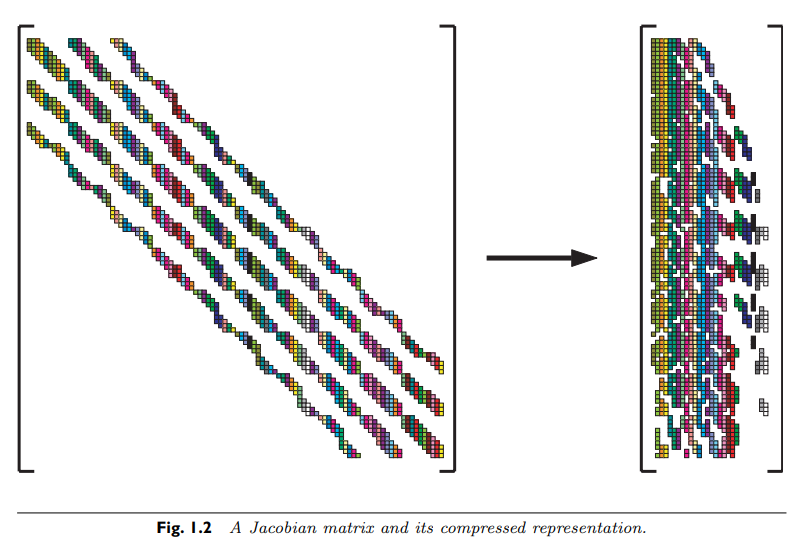With forward-mode automatic differentiation, recall that we calculate multiple dimensions simultaniously by using a multidimensional dual number seeded by the vectors of the differentiation directions, that is:

$d = x + v_1 \epsilon_1 + \ldots + v_m \epsilon_m$

Instead of using the primitive differentiation directions $e_j$, we can instead replace this with the mixed values. For example, the Jacobian of the example function can be computed in one function call to $f$ with the dual number input:

$d = x + (e_1 + e_2) \epsilon_1 + e_3 \epsilon_2$

and performing the decompression via the sparsity pattern. Thus the sparsity pattern gives a direct way to optimize the construction of the Jacobian.

This idea of independent directions can be formalized as a matrix coloring. Take $S_{ij}$ the sparsity pattern of some Jacobian matrix $J_{ij}$. Define a graph on the nodes 1 through m where there is an edge between $i$ and $j$ if there is a row where $i$ and $j$ are non-zero. This graph is the column connectivity graph of the Jacobian. What we wish to do is find the smallest set of differentiation directions such that differentiating in the direction of $e_i$ does not collide with differentiation in the direction of $e_j$. The connectivity graph is setup so that way this cannot be done if the two nodes are adjacent. If we let the subset of nodes differentiated together be a color, the question is, what is the smallest number of colors s.t. no adjacent nodes are the same color. This is the classic distance-1 coloring problem from graph theory. It is well-known that the problem of finding the chromatic number, the minimal number of colors for a graph, is generally NP-complete. However, there are heuristic methods for performing a distance-1 coloring quite quickly. For example, a greedy algorithm is as follows:

• Pick a node at random to be color 1.

• Make all nodes adjacent to that be the lowest color that they can be (in this step that will be 2).

• Now look at all nodes adjacent to that. Make all nodes be the lowest color that they can be (either 1 or 3).

• Repeat by looking at the next set of adjacent nodes and color as conservatively as possible.

This can be visualized as follows: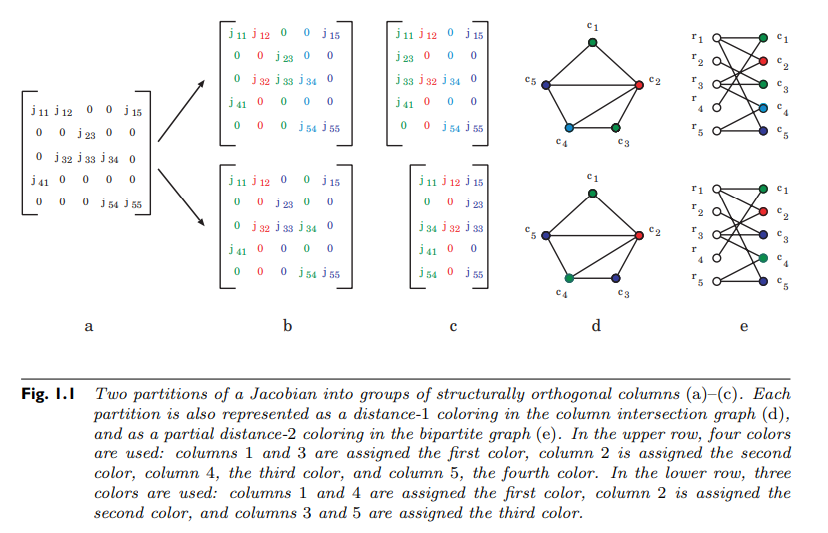The result will color the entire connected component. While not giving an optimal result, it will still give a result that is a sufficient reduction in the number of differentiation directions (without solving an NP-complete problem) and thus can lead to a large computational saving.

At the end, let $c_i$ be the vector of 1's and 0's, where it's 1 for every node that is color $i$ and 0 otherwise. Sparse automatic differentiation of the Jacobian is then computed with:

$d = x + c_1 \epsilon_1 + \ldots + c_k \epsilon_k$

that is, the full Jacobian is computed with one dual number which consists of the primal calculation along with $k$ dual dimensions, where $k$ is the computed chromatic number of the connectivity graph on the Jacobian. Once this calculation is complete, the colored columns can be decompressed into the full Jacobian using the sparsity information, generating the original quantity that we wanted to compute.

For more information on the graph coloring aspects, find the paper titled "What Color Is Your Jacobian? Graph Coloring for Computing Derivatives" by Gebremedhin.

## Jacobian-Free Newton Krylov (JFNK)

An alternative method for solving the linear system is the Jacobian-Free Newton Krylov technique. This technique is broken into two pieces: the jvp calculation and the Krylov subspace iterative linear solver.

### Jacobian-Vector Products as Directional Derivatives

We don't actually need to compute $J$ itself, since all that we actually need is the v = J*w. Is it possible to compute the Jacobian-Vector Product, or the jvp, without producing the Jacobian?

To see how this is done let's take a look at what is actually calculated. Written out in the standard basis, we have that:

$w_i = \sum_{j}^{m} J_{ij} v_{j}$

Now write out what $J$ means and we see that:

$w_i = \sum_j^{m} \frac{df_i}{dx_j} v_j = \nabla f_i(x) \cdot v$

that is, the $i$th component of $Jv$ is the directional derivative of $f_i$ in the direction $v$. This means that in general, the jvp $Jv$ is actually just the directional derivative in the direction of $v$, that is:

$Jv = \nabla f \cdot v$

and therefore it has another mathematical representation, that is:

$Jv = \lim_{\epsilon \rightarrow 0} \frac{f(x+v \epsilon) - f(x)}{\epsilon}$

From this alternative form it is clear that we can always compute a jvp with a single computation. Using finite differences, a simple approximation is the following:

$Jv \approx \frac{f(x+v \epsilon) - f(x)}{\epsilon}$

for non-zero $\epsilon$. Similarly, recall that in forward-mode automatic differentiation we can choose directions by seeding the dual part. Therefore, using the dual number with one partial component:

$d = x + v \epsilon$

we get that

$f(d) = f(x) + Jv \epsilon$

and thus a single application with a single partial gives the jvp.

#### Note on Reverse-Mode Automatic Differentiation

As noted earlier, reverse-mode automatic differentiation has its primitives compute rows of the Jacobian in the seeded direction. This means that the seeded reverse-mode call with the vector $v$ computes $v^T J$, that is the vector (transpose) Jacobian transpose, or vjp for short. When discussing parameter estimation and adjoints, this shorthand will be introduced as a way for using a traditionally machine learning tool to accelerate traditionally scientific computing tasks.

#### Krylov Subspace Methods

While the classical iterative solver methods give the background for understanding an alternative to direct inversion or factorization of a matrix, the problem with that approach is that it requires the ability to split the matrix $J$, which we would like to avoid computing. Instead, we would like to develop an iterative solver technique which instead just uses the solution to $Jv$. Indeed there are such methods, and these are the Krylov subspace methods. A Krylov subspace is the space spanned by:

$\mathcal{K}_k = \text{span} \{v,Jv,J^2 v, \ldots, J^k v\}$

There are a few nice properties about Krylov subspaces that can be exploited. For one, it is known that there is a finite maximum dimension of the Krylov subspace, that is there is a value $r$ such that $J^{r+1} v \in \mathcal{K}_r$, which means that the complete Krylov subspace can be computed in finitely many jvp, since $J^2 v$ is just the jvp where the vector is the jvp. Indeed, one can show that $J^i v$ is linearly independent for each $i$, and thus that maximal value is $m$, the dimension of the Jacobian. Therefore in $m$ jvps the solution is guerenteed to live in the Krylov subspace, giving a maximal computational cost and a proof of convergence if the vector in there is the "optimal in the space".

The most common method in the Krylov subspace family of methods is the GMRES method. Essentially, in step $i$ one computes $\mathcal{K}_i$, and finds the $x$ that is the closest to the Krylov subspace, i.e. finds the $x \in \mathcal{K}_i$ such that $\Vert Jx-v \Vert$ is minimized. At each step, it adds the new vector to the Krylov subspace after orthgonalizing it against the other vectors via Arnoldi iterations, leading to an orthoganol basis of $\mathcal{K}_i$ which makes it easy to express $x$.

While one has a guerenteed bound on the number of possible jvps in GMRES which is simply the number of ODEs (since that is what determines the size of the Jacobian and thus the total dimension of the problem), that bound is not necessarily a good one. For a large sparse matrix, it may be computationally impractical to ever compute 100,000 jvps. Thus one does not typically run the algorithm to conclusion, and instead stops when $\Vert Jx-v \Vert$ is sufficiently below some user-defined error tolerance.

## Working with Stiff ODEs

Let's use this information and switch our brains back over to computing. We will put to use the ideas that we have described here to better enhance some differential equation solving.

### Small Scale ODEs

Let's do some experiments on a canonical stiff ODE, the Robertson equation:

\begin{aligned} \frac{dy_1}{dt} &= -0.04y₁ + 10^4 y_2 y_3 \\ \frac{dy_2}{dt} &= 0.04 y_1 - 10^4 y_2 y_3 - 3*10^7 y_{2}^2 \\ \frac{dy_3}{dt} &= 3*10^7 y_{3}^2 \\ \end{aligned}

This is implemented in DifferentialEquations.jl in the following form:

using OrdinaryDiffEq, Plots
function rober(du,u,p,t)
y₁,y₂,y₃ = u
k₁,k₂,k₃ = p
du = -k₁*y₁+k₃*y₂*y₃
du =  k₁*y₁-k₂*y₂^2-k₃*y₂*y₃
du =  k₂*y₂^2
nothing
end
prob = ODEProblem(rober,[1.0,0.0,0.0],(0.0,1e5),(0.04,3e7,1e4))
sol = solve(prob,Tsit5())
plot(sol,tspan=(1e-2,1e5),xscale=:log10)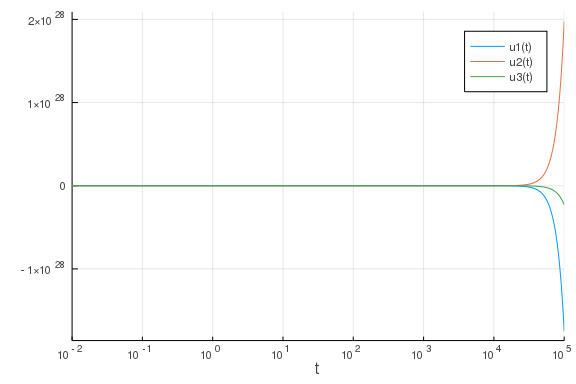This was with an explicit Runge-Kutta method. Notice:

length(sol.t)

999997


which caused an exit. If we instead use a method for stiff equations we can get better results:

sol = solve(prob,Rosenbrock23())
plot(sol,tspan=(1e-2,1e5),xscale=:log10)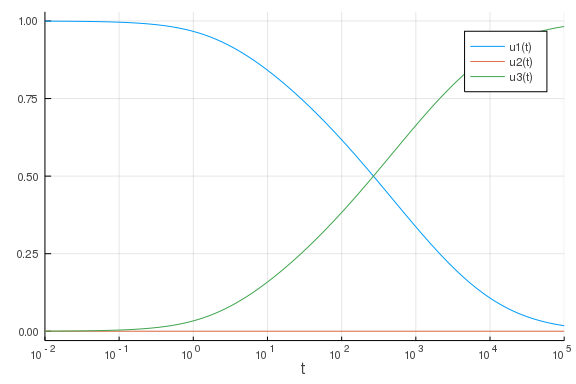One way to notice that this equation is stiff is to recognize that it has some fast terms, given by high reaction rates, mixed with slow terms, given by low reaction rates.

Note that this system can also be written as a differential-algebraic equation with a conservation equation. We can solve this by using and defining a mass matrix formulation:

\begin{aligned} \frac{dy_1}{dt} &= -0.04y₁ + 10^4 y_2 y_3 \\ \frac{dy_2}{dt} &= 0.04 y_1 - 10^4 y_2 y_3 - 3*10^7 y_{2}^2 \\ 1 &= y_{1} + y_{2} + y_{3} \\ \end{aligned}

using OrdinaryDiffEq
function rober_mm(du,u,p,t)
y₁,y₂,y₃ = u
k₁,k₂,k₃ = p
du = -k₁*y₁+k₃*y₂*y₃
du =  k₁*y₁-k₂*y₂^2-k₃*y₂*y₃
du =  y₁ + y₂ + y₃ - 1
nothing
end
M = [1. 0  0
0  1. 0
0  0  0]
f = ODEFunction(rober_mm,mass_matrix=M)
prob_mm = ODEProblem(f,[1.0,0.0,0.0],(0.0,1e5),(0.04,3e7,1e4))
sol = solve(prob_mm,Rodas5(),reltol=1e-8,abstol=1e-8)
plot(sol, xscale=:log10, tspan=(1e-6, 1e5), layout=(3,1))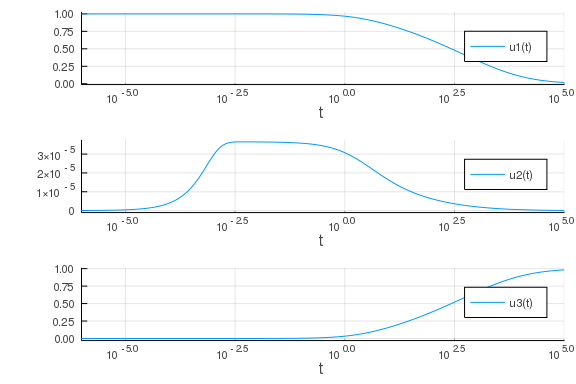While the mass matrix itself is singular, recall that in the Newton iterations we are using the matirx $M-\gamma f^\prime$ which here is not singular, and so the DAE is able to be solved.

Here we can accelerate the solution of this system by writing down the Jacobian:

function rober_jac(J,u,p,t)
y₁,y₂,y₃ = u
k₁,k₂,k₃ = p
J[1,1] = k₁ * -1
J[2,1] = k₁
J[3,1] = 0
J[1,2] = y₃ * k₃
J[2,2] = y₂ * k₂ * -2 + y₃ * k₃ * -1
J[3,2] = y₂ * 2 * k₂
J[1,3] = k₃ * y₂
J[2,3] = k₃ * y₂ * -1
J[3,3] = 0
nothing
end
f = ODEFunction(rober, jac=rober_jac)
prob_jac = ODEProblem(f,[1.0,0.0,0.0],(0.0,1e5),(0.04,3e7,1e4))
sol = solve(prob_jac,Rosenbrock23(),reltol=1e-8,abstol=1e-8)
plot(sol, xscale=:log10, tspan=(1e-6, 1e5), layout=(3,1))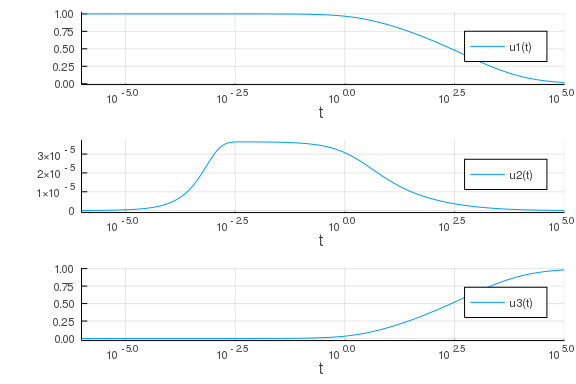using BenchmarkTools
@btime solve(prob,Rodas5(),reltol=1e-8,abstol=1e-8)

591.399 μs (3264 allocations: 201.28 KiB)
retcode: Success
Interpolation: 3rd order Hermite
t: 182-element Array{Float64,1}:
0.0
0.0003332673315028293
0.0004898637281674722
0.0007839051640433667
0.0010143561990243128
0.0013802055458594798
0.001772668192432905
0.0022300817529090026
0.0027415328603086304
0.003359315457308333
⋮
59575.70889414806
64045.5328541224
68882.14696840316
74119.03782189258
79793.06894126732
85944.8995739889
92619.39922028879
99866.1468476492
100000.0
u: 182-element Array{Array{Float64,1},1}:
[1.0, 0.0, 0.0]
[0.9999866694005515, 1.276862592353049e-5, 5.619735248292863e-7]
[0.9999804056741745, 1.7907828328867662e-5, 1.686497496552089e-6]
[0.9999686445547237, 2.5399362314060852e-5, 5.956082962307945e-6]
[0.9999594273846907, 2.9374337954534597e-5, 1.1198277355102703e-5]
[0.9999447960145582, 3.312692595101817e-5, 2.2077059490894208e-5]
[0.9999291023792589, 3.503814576582433e-5, 3.5859474975353634e-5]
[0.9999108145430978, 3.596001621540818e-5, 5.322544068686379e-5]
[0.9998903702348204, 3.6324450365362516e-5, 7.330531481426154e-5]
[0.9998656811910945, 3.645296904143272e-5, 9.786583986406176e-5]
⋮
[0.028019157655499994, 1.152664539488619e-7, 0.9719807270780435]
[0.02634677498079004, 1.0820278261108894e-7, 0.9736531168164244]
[0.024753361388615024, 1.0149489208198633e-7, 0.9752465371164899]
[0.02323652848048233, 9.512945247205468e-8, 0.9767633763900626]
[0.021793888246710122, 8.9093440581567e-8, 0.978206022659847]
[0.020423046359218218, 8.337409169105537e-8, 0.979576870266688]
[0.01912161179451773, 7.795892076429868e-8, 0.9808783102465594]
[0.017887198646191312, 7.283571167064268e-8, 0.9821127285180958]
[0.01786592163629542, 7.274751673280214e-8, 0.9821340056161866]

@btime solve(prob_jac,Rodas5(),reltol=1e-8,abstol=1e-8)

387.201 μs (3064 allocations: 185.19 KiB)
retcode: Success
Interpolation: 3rd order Hermite
t: 182-element Array{Float64,1}:
0.0
0.0003332673315028293
0.0004898637281674722
0.0007839051640433667
0.0010143561990243128
0.0013802055458594798
0.001772668192432905
0.0022300817529090026
0.0027415328603086304
0.003359315457308333
⋮
59575.70889414806
64045.5328541224
68882.14696840316
74119.03782189258
79793.06894126732
85944.8995739889
92619.39922028879
99866.1468476492
100000.0
u: 182-element Array{Array{Float64,1},1}:
[1.0, 0.0, 0.0]
[0.9999866694005515, 1.276862592353049e-5, 5.619735248292863e-7]
[0.9999804056741745, 1.7907828328867662e-5, 1.686497496552089e-6]
[0.9999686445547237, 2.5399362314060852e-5, 5.956082962307945e-6]
[0.9999594273846907, 2.9374337954534597e-5, 1.1198277355102703e-5]
[0.9999447960145582, 3.312692595101817e-5, 2.2077059490894208e-5]
[0.9999291023792589, 3.503814576582433e-5, 3.5859474975353634e-5]
[0.9999108145430978, 3.596001621540818e-5, 5.322544068686379e-5]
[0.9998903702348204, 3.6324450365362516e-5, 7.330531481426154e-5]
[0.9998656811910945, 3.645296904143272e-5, 9.786583986406176e-5]
⋮
[0.028019157655499994, 1.152664539488619e-7, 0.9719807270780435]
[0.02634677498079004, 1.0820278261108894e-7, 0.9736531168164244]
[0.024753361388615024, 1.0149489208198633e-7, 0.9752465371164899]
[0.02323652848048233, 9.512945247205468e-8, 0.9767633763900626]
[0.021793888246710122, 8.9093440581567e-8, 0.978206022659847]
[0.020423046359218218, 8.337409169105537e-8, 0.979576870266688]
[0.01912161179451773, 7.795892076429868e-8, 0.9808783102465594]
[0.017887198646191312, 7.283571167064268e-8, 0.9821127285180958]
[0.01786592163629542, 7.274751673280214e-8, 0.9821340056161866]


Instead of writing the Jacobian itself, we can use Julia program transformation tooling to generate the Jacobian. Here we will showcase ModelingToolkit.jl. There is an automatic way via modelingtoolkitize:

using ModelingToolkit
de = modelingtoolkitize(prob)
ModelingToolkit.generate_jacobian(de...)

:((var"##MTIIPVar#559", u, p, t)->begin
#= C:\Users\accou\.julia\packages\ModelingToolkit\5O0vP\src\utils
.jl:77 =#
#= C:\Users\accou\.julia\packages\ModelingToolkit\5O0vP\src\utils
.jl:78 =#
#= C:\Users\accou\.julia\packages\ModelingToolkit\5O0vP\src\utils
.jl:78 =# @inbounds begin
#= C:\Users\accou\.julia\packages\ModelingToolkit\5O0vP\s
rc\utils.jl:79 =#
#= C:\Users\accou\.julia\packages\ModelingToolkit\5O0vP\s
rc\utils.jl:58 =# @inbounds begin
#= C:\Users\accou\.julia\packages\ModelingToolkit
\5O0vP\src\utils.jl:58 =#
let (x₁, x₂, x₃, α₁, α₂, α₃) = (u, u, u,
p, p, p)
var"##MTIIPVar#559" = α₁ * -1
var"##MTIIPVar#559" = α₁
var"##MTIIPVar#559" = 0
var"##MTIIPVar#559" = x₃ * α₃
var"##MTIIPVar#559" = x₂ * α₂ * -2 + x₃ *
α₃ * -1
var"##MTIIPVar#559" = x₂ * 2 * α₂
var"##MTIIPVar#559" = α₃ * x₂
var"##MTIIPVar#559" = α₃ * x₂ * -1
var"##MTIIPVar#559" = 0
end
end
end
#= C:\Users\accou\.julia\packages\ModelingToolkit\5O0vP\src\utils
.jl:81 =#
nothing
end)


We can also do this more manually. Under the hood, this is using multiple dispatch to trace the computational graph of our program and then perform symbolic operations to understand the system.

using ModelingToolkit
@variables y[1:3] t
@parameters p[1:3]

y + y^2

dy = copy(y)
rober(dy,y,p,t)
dy

3-element Array{ModelingToolkit.Operation,1}:
-p₁ * y₁ + (p₃ * y₂) * y₃
(p₁ * y₁ - p₂ * y₂ ^ 2) - (p₃ * y₂) * y₃
p₂ * y₂ ^ 2


which is now a symbolic form and the ModelingToolkit.jl library can be utilized to transform it however needed.

### Large Sparse ODEs and Partial Differential Equations

The classic case of a large ODE that is stiff is a discretization of a partial differential equation. Let's do a bit of theory first to learn why PDEs are stiff.

#### Von Neumann Analysis of Heat Equation

Let's take a look at Von Neumann analysis of PDE stability.

Let's look at the error update equation. Write

$e_{i}^{n}=u(x_{j},t_{n})-u_{j}^{n}$

For $e_{i}^{n}$, as before, plug it in, add and subtract $u(x_{j},t^{n})=u_{j}^{n}$, and then we get

$e_{i}^{n+1}=e_{i}^{n}+\mu\left(e_{i+1}^{n}-2e_{i}^{n}+e_{i-1}^{n}\right)+\Delta t\tau_{i}^{n}$

where:

$\mu = \frac{\Delta t}{\Delta x^2}$

$\tau_{i}^{n}\sim\mathcal{O}(\Delta t)+\mathcal{O}(\Delta x^{2})$

.

Stability requires that the homogenous equation goes to zero. Another way of saying that is that the propogation of errors has errors decrease their influence over time. Thus we look at:

$e_{i}^{n+1} =e_{i}^{n}+\mu\left(e_{i+1}^{n}-2e_{i}^{n}+e_{i-1}^{n}\right) =\left(1-2\mu\right)e_{i}^{n}+\mu e_{i+1}^{n}+\mu e_{i-1}^{n}$

A necessary condition for decreasing is then for all coefficients to be positive

$1-2\mu\geq0$

or

$\mu\leq\frac{1}{2}$

A more satisfying way may be to look at the generated ODE

$u^{\prime}=Au$

where A is the matrix $\left[\mu,1-2\mu,\mu\right].$

But finding the maximum eigenvalue is non-trivial. But for linear PDEs, one nice way to analyze the stability directly is to use the Fourier mode decomposition. This is known as Van Neumann stability analysis. To do this, decompose $U$ into the Fourier modes:

$U(x,t)=\sum_{k}\hat{U}(t)e^{ikx}$

Since

$x_{j}=j\Delta x,$

we can write this out as

$U_{j}^{n}=\hat{U}^{n}e^{ikj\Delta x}$

and then plugging this into the FTCS scheme we get

$\frac{\hat{U}^{n+1}e^{ikj\Delta x}-\hat{U}^{n}e^{ikj\Delta x}}{\Delta t}=\frac{\hat{U}^{n}e^{ik(j+1)\Delta x}-2\hat{U}^{n}e^{ikj\Delta x}+\hat{U}^{n}e^{ik(j-1)\Delta x}}{\Delta x^{2}}$

Let G be the growth factor, defined as

$G=\frac{\hat{U}^{n+1}}{\hat{U}^{n}}$

and thus after cancelling we get

$\frac{G-1}{\Delta t}=\frac{e^{ik\Delta x}-2+e^{-ik\Delta x}}{\Delta x^{2}}$

Since

$e^{ik\Delta x}+e^{-ik\Delta x}=2\cos\left(k\Delta x\right),$

then we get

$G=1-\mu\left(2\cos\left(k\Delta x\right)-2\right)$

and using the half angle formula

$G=1-4\mu\sin^{2}\left(\frac{k\Delta x}{2}\right)$

In order to be stable, we require

$\left|G\right|\leq1,$

which means

$-1\leq1-4\mu\sin^{2}\left(\frac{k\Delta x}{2}\right)\leq1 since \mu>0 (since \Delta t>0 and \Delta x > 0) and so \leq1 is simple. Since \sin^{2}(x)\leq1, then we can simplify this to -1\leq1-4\mu$

and thus $\mu\leq\frac{1}{2}$. With backwards Euler we get

$\frac{G-1}{\Delta t}=\frac{G}{\Delta x^{2}}\left(e^{ik\Delta x}-2+e^{-ik\Delta x}\right)$

and thus get

$G+4G\mu\sin^{2}\left(\frac{k\Delta x}{2}\right)=1$

and thus

$G=\frac{1}{1+4\mu\sin^{2}\left(\frac{k\Delta x}{2}\right)}\leq1.$

$\mu>0$

and so $\leq 1$ is simple. Since $\sin^{2}(x)\leq 1$, then we can simplify this to:

$-1 \leq 1-4 \mu$

and thus $\mu\leq\frac{1}{2}$. With backwards Euler we get:

$\frac{G-1}{\Delta t}=\frac{G}{\Delta x^{2}}\left(e^{ik\Delta x}-2+e^{-ik\Delta x}\right)$

and thus get

$G+4G\mu\sin^{2}\left(\frac{k\Delta x}{2}\right)=1$

and thus

$G=\frac{1}{1+4\mu\sin^{2}\left(\frac{k\Delta x}{2}\right)}\leq 1.$

This goes to show that explicit methods impose a stepsize restriction on PDEs while implicit methods do not!

#### Handling the Brusselator

Let's take a look at the Brusselator equation:

$\frac{\partial u}{\partial t} =1+u^{2}v-4.4u+\alpha\Delta u+f(x,y,t)$

$\frac{\partial v}{\partial t} =3.4u-u^{2}v+\alpha\Delta v$

with

$\Delta x=\Delta y=\frac{1}{32},$

$f(x,y,t)=\begin{cases} 5 & (x-0.3)^{2}+(y-0.6)^{2}\leq 0.1^{2}\,\,\,\&\,\,\,t\geq1.1\\ 0 & o.w. \end{cases}$

and initial conditions

$u(x,0)=22y(1-y)^{\frac{3}{2}}$

$v(x,0) =27x(1-x)^{\frac{3}{2}}$

with periodic boundary conditions

$u(x,t) =u(x+1,t)$

$u(y,t) =u(y+1,t)$

$v(x,t) =v(x+1,t)$

$v(y,t) =v(y+1,t)$

and parameters

$A =3.4$

$B =1.0$

$\alpha =10.0$

Using the method shown in the previous class, this becomes the ODE code as follows:

const N = 32
const xyd_brusselator = range(0,stop=1,length=N)
brusselator_f(x, y, t) = (((x-0.3)^2 + (y-0.6)^2) <= 0.1^2) * (t >= 1.1) * 5.
limit(a, N) = a == N+1 ? 1 : a == 0 ? N : a
function brusselator_2d_loop(du, u, p, t)
A, B, alpha, dx = p
alpha = alpha/dx^2
@inbounds for I in CartesianIndices((N, N))
i, j = Tuple(I)
x, y = xyd_brusselator[I], xyd_brusselator[I]
ip1, im1, jp1, jm1 = limit(i+1, N), limit(i-1, N), limit(j+1, N), limit(j-1, N)
du[i,j,1] = alpha*(u[im1,j,1] + u[ip1,j,1] + u[i,jp1,1] + u[i,jm1,1] - 4u[i,j,1]) +
B + u[i,j,1]^2*u[i,j,2] - (A + 1)*u[i,j,1] + brusselator_f(x, y, t)
du[i,j,2] = alpha*(u[im1,j,2] + u[ip1,j,2] + u[i,jp1,2] + u[i,jm1,2] - 4u[i,j,2]) +
A*u[i,j,1] - u[i,j,1]^2*u[i,j,2]
end
end
p = (3.4, 1., 10., step(xyd_brusselator))

(3.4, 1.0, 10.0, 0.03225806451612903)


To get the sparsity pattern

using SparsityDetection, SparseArrays
input = rand(32,32,2)
output = similar(input)
sparsity_pattern = sparsity!(brusselator_2d_loop,output,input,p,0.0)

Explored path: SparsityDetection.Path(Bool[], 1)

jac_sparsity = Float64.(sparse(sparsity_pattern))

2048×2048 SparseArrays.SparseMatrixCSC{Float64,Int64} with 12288 stored ent
ries:
[1   ,    1]  =  1.0
[2   ,    1]  =  1.0
[32  ,    1]  =  1.0
[33  ,    1]  =  1.0
[993 ,    1]  =  1.0
[1025,    1]  =  1.0
[1   ,    2]  =  1.0
[2   ,    2]  =  1.0
[3   ,    2]  =  1.0
⋮
[2015, 2047]  =  1.0
[2046, 2047]  =  1.0
[2047, 2047]  =  1.0
[2048, 2047]  =  1.0
[1024, 2048]  =  1.0
[1056, 2048]  =  1.0
[2016, 2048]  =  1.0
[2017, 2048]  =  1.0
[2047, 2048]  =  1.0
[2048, 2048]  =  1.0

using Plots
spy(jac_sparsity,markersize=1,colorbar=false,color=:deep)We can now define an ODEFunction that utilizes the sparsity pattern:

ff = ODEFunction(brusselator_2d_loop;jac_prototype=jac_sparsity)

(::DiffEqBase.ODEFunction{true,typeof(Main.WeaveSandBox1.brusselator_2d_loo
p),LinearAlgebra.UniformScaling{Bool},Nothing,Nothing,Nothing,Nothing,Nothi
ng,SparseArrays.SparseMatrixCSC{Float64,Int64},Nothing,Nothing,Nothing,Noth
ing,Nothing}) (generic function with 7 methods)


and now setup the Brusselator problem with both the sparse and non-sparse versions:

function init_brusselator_2d(xyd)
N = length(xyd)
u = zeros(N, N, 2)
for I in CartesianIndices((N, N))
x = xyd[I]
y = xyd[I]
u[I,1] = 22*(y*(1-y))^(3/2)
u[I,2] = 27*(x*(1-x))^(3/2)
end
u
end
u0 = init_brusselator_2d(xyd_brusselator)
prob_ode_brusselator_2d = ODEProblem(brusselator_2d_loop,
u0,(0.,11.5),p)

prob_ode_brusselator_2d_sparse = ODEProblem(ff,
u0,(0.,11.5),p)

ODEProblem with uType Array{Float64,3} and tType Float64. In-place: true
timespan: (0.0, 11.5)
u0: [0.0 0.12134432813715876 … 0.1213443281371586 0.0; 0.0 0.12134432813715
876 … 0.1213443281371586 0.0; … ; 0.0 0.12134432813715876 … 0.1213443281371
586 0.0; 0.0 0.12134432813715876 … 0.1213443281371586 0.0]

[0.0 0.0 … 0.0 0.0; 0.14892258453196755 0.14892258453196755 … 0.14892258453
196755 0.14892258453196755; … ; 0.14892258453196738 0.14892258453196738 … 0
.14892258453196738 0.14892258453196738; 0.0 0.0 … 0.0 0.0]


and see the speed difference:

using BenchmarkTools
@btime solve(prob_ode_brusselator_2d,Rodas5(),save_everystep=false)

7.653 s (1332929 allocations: 138.06 MiB)

@btime solve(prob_ode_brusselator_2d_sparse,Rodas5(),save_everystep=false)

4.868 s (1488255 allocations: 491.07 MiB)
retcode: Success
Interpolation: 1st order linear
t: 2-element Array{Float64,1}:
0.0
11.5
u: 2-element Array{Array{Float64,3},1}:
[0.0 0.12134432813715876 … 0.1213443281371586 0.0; 0.0 0.12134432813715876
… 0.1213443281371586 0.0; … ; 0.0 0.12134432813715876 … 0.1213443281371586
0.0; 0.0 0.12134432813715876 … 0.1213443281371586 0.0]

[0.0 0.0 … 0.0 0.0; 0.14892258453196755 0.14892258453196755 … 0.14892258453
196755 0.14892258453196755; … ; 0.14892258453196738 0.14892258453196738 … 0
.14892258453196738 0.14892258453196738; 0.0 0.0 … 0.0 0.0]

[3.3016585314010465 3.301631318912525 … 3.3017494393530997 3.3016981231782
87; 3.301707332996093 3.3016768503238474 … 3.3018098420441238 3.30175184727
3377; … ; 3.301573167306625 3.301551261765275 … 3.3016454696182373 3.301604
817907413; 3.3016133156855183 3.301588978758814 … 3.301694110690706 3.30164
85979949612]

[2.2650413719148483 2.2650427939218853 … 2.2650367337118578 2.2650393315964
723; 2.265038540333992 2.2650400668506085 … 2.265033547797888 2.26503634669
48735; … ; 2.2650464161765047 2.265047661577886 … 2.2650423745253234 2.2650
446345861863; 2.2650440292824205 2.265045356733698 … 2.265039710744344 2.26
50421275206445]


This isn't all that much, but that's because we haven't specialized on the color patterns yet! We can use SparseDiffTools.jl in order to color the Jacobian given it's sparsity pattern via its matrix_colors function.

using SparseDiffTools
colorvec = matrix_colors(jac_sparsity)
@show maximum(colorvec)

maximum(colorvec) = 12
12

colorvec

2048-element Array{Int64,1}:
1
2
3
1
2
3
1
2
3
1
⋮
11
9
10
11
9
10
4
5
10


This shows that we only need to do 12 f calls to build the Jacobian (instead of 2048!). We can now pass the colorvec to the ODEFunction to specialize the Jacobian construction and see a massive speedup:

ff2 = ODEFunction(brusselator_2d_loop;jac_prototype=jac_sparsity,
colorvec=colorvec)
prob_ode_brusselator_2d_sparse = ODEProblem(ff2,
init_brusselator_2d(xyd_brusselator),
(0.,11.5),p)
@btime solve(prob_ode_brusselator_2d_sparse,Rodas5(),save_everystep=false)

1.145 s (17517 allocations: 412.79 MiB)
retcode: Success
Interpolation: 1st order linear
t: 2-element Array{Float64,1}:
0.0
11.5
u: 2-element Array{Array{Float64,3},1}:
[0.0 0.12134432813715876 … 0.1213443281371586 0.0; 0.0 0.12134432813715876
… 0.1213443281371586 0.0; … ; 0.0 0.12134432813715876 … 0.1213443281371586
0.0; 0.0 0.12134432813715876 … 0.1213443281371586 0.0]

[0.0 0.0 … 0.0 0.0; 0.14892258453196755 0.14892258453196755 … 0.14892258453
196755 0.14892258453196755; … ; 0.14892258453196738 0.14892258453196738 … 0
.14892258453196738 0.14892258453196738; 0.0 0.0 … 0.0 0.0]

[3.3016585314010465 3.301631318912525 … 3.3017494393530997 3.3016981231782
87; 3.301707332996093 3.3016768503238474 … 3.3018098420441238 3.30175184727
3377; … ; 3.301573167306625 3.301551261765275 … 3.3016454696182373 3.301604
817907413; 3.3016133156855183 3.301588978758814 … 3.301694110690706 3.30164
85979949612]

[2.2650413719148483 2.2650427939218853 … 2.2650367337118578 2.2650393315964
723; 2.265038540333992 2.2650400668506085 … 2.265033547797888 2.26503634669
48735; … ; 2.2650464161765047 2.265047661577886 … 2.2650423745253234 2.2650
446345861863; 2.2650440292824205 2.265045356733698 … 2.265039710744344 2.26
50421275206445]